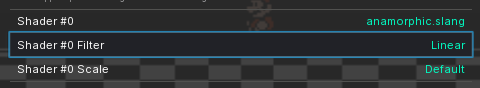# Is it possible to use two presets together?

I recently discovered that I could use two Shaders together, I had tried before and it gave me a lot of errors, because I was doing it wrong, I loaded a .slangp, added a Shader Passes and loaded a .slang.

My confusion was in not knowing how it works and the similarity of the names. This is very interesting because I can shader like I’m putting together a lego.

But I want something a little more advanced, I would like to make a mini preset of the Integer Scaline of the guest shader and then load it together with another preset.

Is it possible to make a preset that loads two presets?

1 Like

You can use the append/prepend functions to combine them serially. If you want to take bits and pieces of different ones, though, you’ll still need to get your hands dirty with a text editor.

1 Like

this is the part I like the least, when you see those files you see shader, I see this.

I’m going to search in the shader for that function to see how it works, if I don’t get it I’ll come back : )

I was experimenting with the anamorphic shader and saw this, shaders such asAnamorphic, Integer Scaling de gues, can be useful to combine with other shaders. The Mega Bezel shader seems to be much more complicated.

By the way, when loaded as a preset, it loads a bilinear filter. When loaded as a shader it doesn’t, looks pixel hard.
Until yesterday, I think, I could place a shader and activate down Bilinear and apply. Now no, it goes back to Default.

Is it really that easy?

I was reading the files, trying to understand how it worked.It works well with basic and in many advanced presets, but in other cases it looks very bad. I tried to merge anamorphic with scalerfx and the result is ugly.

If I load the anamorphic preset the filter is active, if I load the shader it is not, and it is not possible to activate it.
Every time I load a shader or an extra preset, the linear filter is deactivated and I can’t activate it.
Is this behavior normal? maybe I am confused, but I think I remember that before it was updated I could activate it.

1 Like

You should be able to change the filtering using the ‘filter’ field for the pass(es).

It does not change. When I load the preset, Linear is activated. If I load the independent shader, Default is activated.Now I realize, I can’t change it either way, I can’t select Linear or Nearest, because it always returns to Default, when I apply the changes.

Shader #0 Scale, if I can change it.

Probably best to change it in the preset, then.

It seems mixing anamorphic with stuff is a bit wonky because it needs the whole viewport to properly do its effect, so it either needs to be the last pass (which gets scaled to the viewport implicitly) or it needs viewport scaling specified in the shader preset. crt-guest usually wants low-res inputs, but you can use the “hd” flavor of it and then set the internal resolution to where it looks correct (around 5.5-6.0 looked right on my 1080p screen):

``````shaders = 12

textures = "SamplerLUT1;SamplerLUT2;SamplerLUT3;SamplerLUT4"
SamplerLUT1_linear = true
SamplerLUT2_linear = true
SamplerLUT3_linear = true
SamplerLUT4_linear = true

filter_linear0 = false
scale_type0 = source
scale0 = 1.0

filter_linear1 = false
scale_type1 = viewport
scale1 = 1.0
alias1 = StockPass

filter_linear2 = false
scale_type2 = source
scale2 = 1.0
alias2 = AfterglowPass

filter_linear3 = false
scale_type3 = source
mipmap_input3 = true
scale3 = 1.0
alias3 = PrePass

filter_linear4 = true
scale_type4 = source
scale4 = 1.0
float_framebuffer4 = true
alias4 = LinearizePass

filter_linear5 = true
scale_type_x5 = viewport
scale_x5 = 1.0
scale_type_y5 = source
scale_y5 = 1.0
float_framebuffer5 = true
alias5 = Pass1

filter_linear6 = true
scale_type_x6 = absolute
scale_x6 = 800.0
scale_type_y6 = source
scale_y6 = 1.0
float_framebuffer6 = true

filter_linear7 = true
scale_type_x7 = absolute
scale_x7 = 800.0
scale_type_y7 = absolute
scale_y7 = 600.0
float_framebuffer7 = true
alias7 = GlowPass

filter_linear8 = true
scale_type_x8 = absolute
scale_x8 = 800.0
scale_type_y8 = source
scale_y8 = 1.0
float_framebuffer8 = true

filter_linear9 = true
scale_type_x9 = source
scale_x9 = 1.0
scale_type_y9 = absolute
scale_y9 = 600.0
float_framebuffer9 = true
alias9 = BloomPass

filter_linear10 = true
float_framebuffer10 = true
scale_type10 = viewport
scale_x10 = 1.0
scale_y10 = 1.0